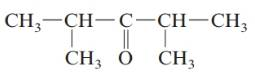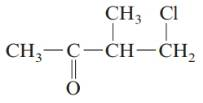Chapter 20, Problem 73QAP### Introductory Chemistry: A Foundati...

9th Edition
Steven S. Zumdahl + 1 other
ISBN: 9781337399425

#### Solutions

Chapter
Section### Introductory Chemistry: A Foundati...

9th Edition
Steven S. Zumdahl + 1 other
ISBN: 9781337399425
Textbook Problem
1 views

# . Give the systematic name for each of the following aldehydes or ketones. a.b.c.d.Interpretation Introduction

Interpretation:

The systematic name of the given aldehyde or ketone should be determined.

Concept Introduction:

The group that contains carboxyl group which is attached to at least one hydrogen is said to be an aldehyde group, general representation of an aldehyde group is RCH=O or RCHO. Whereas the group that contains carboxyl group which is attached to two carbon atoms is said to be a ketone group, general representation of a ketone group is RCOR’.

In order to give the IUPAC name to the aldehyde group, the following steps are followed:

1. The parent (longest) alkane chain is identified.

2. The ending of the parent chain from alkane (-e) is changed to -al for an aldehyde group. The carbonyl group of an aldehyde appear at the end of the carbon chain so, the numbering start with carbon having aldehyde group.

3. Name should be written in alphabetical order and other substituents are shown by the number.

In order to give the IUPAC name to the ketone group, the following steps are followed:

1. The parent (longest) alkane chain is identified.

2. The ending of the parent chain from alkane (-e) is changed to -one for a ketone group.

3. The numbering is of the chain is done in such a way that carbonyl carbon gets the smaller number.

4. Name should be written in alphabetical order and other substituents are shown by the number.

Explanation

The given structure is:

The parent chain in the given structure is pentane. Numbering is done in such a way that carbonyl carbon ge...

Interpretation Introduction

Interpretation:

The systematic name of the given aldehyde or ketone should be determined.

Concept Introduction:

The group that contains carboxyl group which is attached to at least one hydrogen is said to be an aldehyde group, general representation of an aldehyde group is RCH=O or RCHO. Whereas the group that contains carboxyl group which is attached to two carbon atoms is said to be a ketone group, general representation of a ketone group is RCOR’.

In order to give the IUPAC name to the aldehyde group, the following steps are followed:

1. The parent (longest) alkane chain is identified.

2. The ending of the parent chain from alkane (-e) is changed to -al for an aldehyde group. The carbonyl group of an aldehyde appear at the end of the carbon chain so, the numbering start with carbon having aldehyde group.

3. Name should be written in alphabetical order and other substituents are shown by the number.

In order to give the IUPAC name to the ketone group, the following steps are followed:

1. The parent (longest) alkane chain is identified.

2. The ending of the parent chain from alkane (-e) is changed to -one for a ketone group.

3. The numbering is of the chain is done in such a way that carbonyl carbon gets the smaller number.

4. Name should be written in alphabetical order and other substituents are shown by the number.

Interpretation Introduction

Interpretation:

The systematic name of the given aldehyde or ketone should be determined.

Concept Introduction:

The group that contains carboxyl group which is attached to at least one hydrogen is said to be an aldehyde group, general representation of an aldehyde group is RCH=O or RCHO. Whereas the group that contains carboxyl group which is attached to two carbon atoms is said to be a ketone group, general representation of a ketone group is RCOR’.

In order to give the IUPAC name to the aldehyde group, the following steps are followed:

1. The parent (longest) alkane chain is identified.

2. The ending of the parent chain from alkane (-e) is changed to -al for an aldehyde group. The carbonyl group of an aldehyde appear at the end of the carbon chain so, the numbering start with carbon having aldehyde group.

3. Name should be written in alphabetical order and other substituents are shown by the number.

In order to give the IUPAC name to the ketone group, the following steps are followed:

1. The parent (longest) alkane chain is identified.

2. The ending of the parent chain from alkane (-e) is changed to -one for a ketone group.

3. The numbering is of the chain is done in such a way that carbonyl carbon gets the smaller number.

4. Name should be written in alphabetical order and other substituents are shown by the number.

Interpretation Introduction

Interpretation:

The systematic name of the given aldehyde or ketone should be determined.

Concept Introduction:

The group that contains carboxyl group which is attached to at least one hydrogen is said to be an aldehyde group, general representation of an aldehyde group is RCH=O or RCHO. Whereas the group that contains carboxyl group which is attached to two carbon atoms is said to be a ketone group, general representation of a ketone group is RCOR’.

In order to give the IUPAC name to the aldehyde group, the following steps are followed:

1. The parent (longest) alkane chain is identified.

2. The ending of the parent chain from alkane (-e) is changed to -al for an aldehyde group. The carbonyl group of an aldehyde appear at the end of the carbon chain so, the numbering start with carbon having aldehyde group.

3. Name should be written in alphabetical order and other substituents are shown by the number.

In order to give the IUPAC name to the ketone group, the following steps are followed:

1. The parent (longest) alkane chain is identified.

2. The ending of the parent chain from alkane (-e) is changed to -one for a ketone group.

3. The numbering is of the chain is done in such a way that carbonyl carbon gets the smaller number.

4. Name should be written in alphabetical order and other substituents are shown by the number.

### Still sussing out bartleby?

Check out a sample textbook solution.

See a sample solution

#### The Solution to Your Study Problems

Bartleby provides explanations to thousands of textbook problems written by our experts, many with advanced degrees!

Get Started

#### Find more solutions based on key concepts